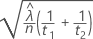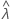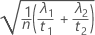# Methods and formulas for Power and Sample Size for 2-Sample Poisson Rate

Select the method or formula of your choice.

## Calculating power

### Formula

One-sided power ( H 1: λ1 > λ2)

One-sided power ( H 1: λ1 < λ2)

Two-sided power ( H 1: λ1λ2)

### Notation

TermDescription
Φ the cumulative distribution function (CDF) of the standard normal distribution
z αΦ -1 (1- α) where Φ -1 is the inverse CDF of the standard normal distribution
λ 1the comparison rate
λ 2the baseline rate
σ 0standard deviation under null hypothesis:σ Astandard deviation under alternative hypothesis:t 1length for group 1
t 2length for group 2

## Calculating sample size and comparison rate

If you provide values for power and sample size, Minitab calculates the value of the comparison rate. If you provide values for power and comparison rate, Minitab calculates the value of the sample size.

For these two cases, Minitab uses an iterative algorithm to find the optimal value. At each iteration, Minitab evaluates the power for a trial sample size or trial comparison rate, and stops when the power function equals a target power value.

### Target power and actual power

When Minitab calculates sample size, it may find that no integer value of sample size yields your target power. In such cases, Minitab displays the target value for power alongside the actual power, which is a value corresponding to an integer sample size, and which is nearest to, yet greater than, the target value.

By using this site you agree to the use of cookies for analytics and personalized content.  Read our policy